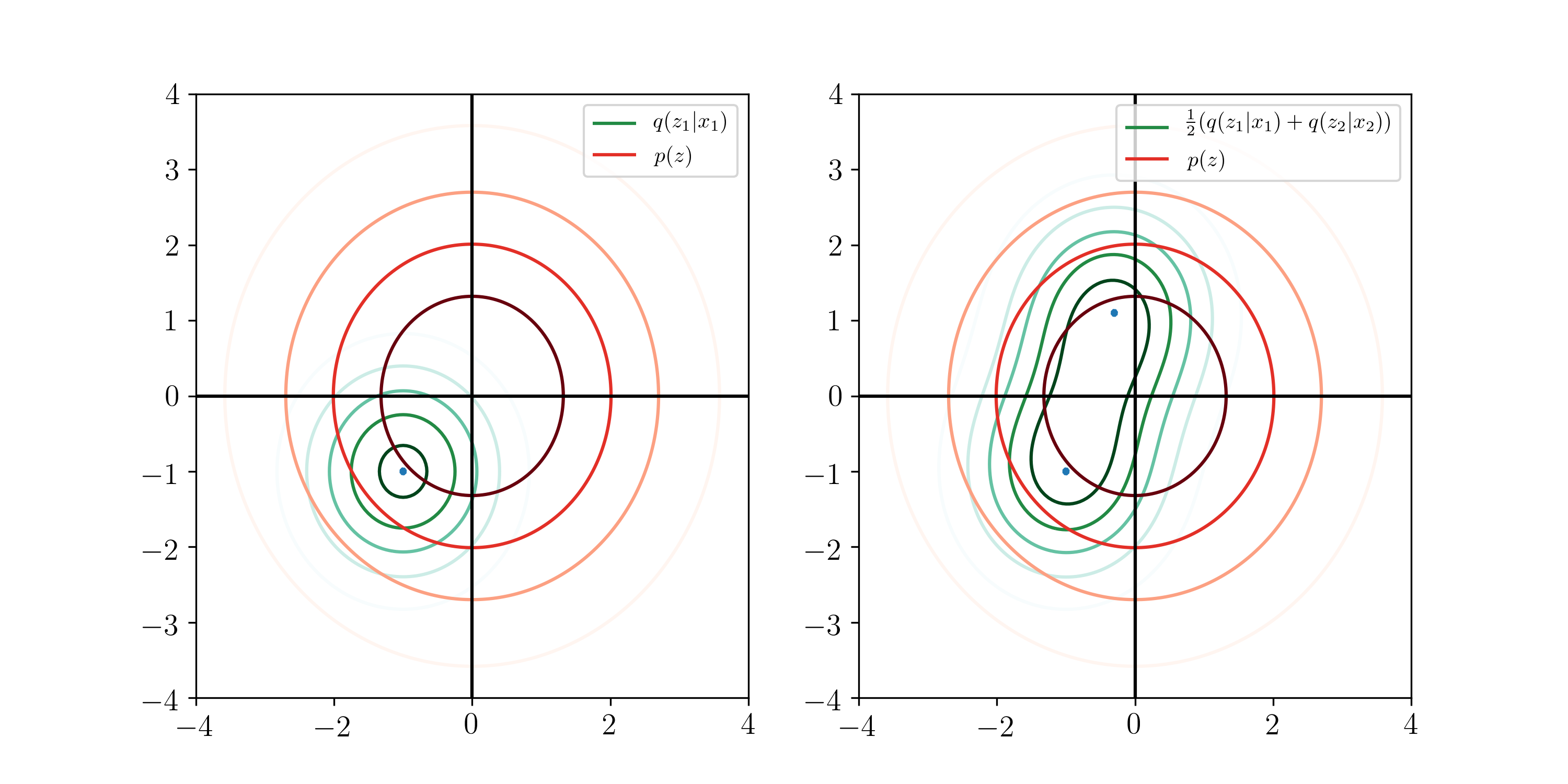# ELBO Surgery

December 23, 2017

tldr: The ubiquitous isotropic Gaussian prior for generative models doesn't make sense / doesn't work, which motivates work on priors.

At NIPS, Dawen Liang mentioned Hoffman & Johnson's ELBO surgery paper offhand while talking about tuning KL divergences, and it's very interesting, so I thought I'd go over it. It's very clearly written, so I won't go into any of the derivations, but instead offer my interpretation.

### Motivation

I worked in the past on applying variational inference and comparing it to models trained via MAP/MLE inference. I decomposed the evidence lower bound (ELBO) as:

$$\mathcal{L} = \frac{1}{N}\sum_{i = 1}^N\left(\underbrace{\mathbb{E}_{q(z_n | x_n)}[\log p(x_n | z_n)]}_{\text{log-likelihood}} - \underbrace{\operatorname{KL}(q(z_n | x_n) || p(z_n))}_{\text{KL divergence}}\right)$$

This is, I think, the most common interpretation: split the ELBO into a reconstruction term and a KL divergence term. The first encourages the model to reconstruct the data, and the second regularizes the model, asking the posterior distribution over $z_n$ to have a certain shape, like a Gaussian. For example, in a VAE the second term is what prevents the model from just learning a Dirac delta-like posterior $q(z_n | x_n) \sim \mathcal{N}(x_n, 0.001)$ around the original value1.

In the NLP world, people have seen some problems though - when we have a very powerful generative model (e.g., an RNN), the KL divergence can vanish. This means the posterior $q(z_n | x_n) \approx p(z_n)$ learns nothing about the data, so the generative model $p(x_n | z_n)$ becomes like a language model. The usual trick is to anneal the KL divergence term in, so that that inference can be useful. A lot of people are unhappy with this because it adds extra hyperparameters and it feels really non-Bayesian.

### Contribution

The contribution of this paper is the following observation: the KL divergence above measures the distance from the posterior for a single $z_n$ to the prior, but we really care about the KL divergence from the average posterior over all data points to the prior. So they define

$$q(z) = \frac{1}{N}\sum_{n = 1}^Nq(z_n | x_n)$$

which is the average posterior we see. The intuition here is that when we're trying to do inference, we shouldn't exactly be penalized for being very confident in $q(z_n | x_n)$. However, we want the average distribution to be close to the prior, so this term can go to 0 safely without worrying about whether the posterior has learned something. In fact, at the cost of a lot of extra computation, we can even safely set the prior to be this distribution, or let $p(z) \triangleq q(z)$!Then, they view $n$, the index variable, as a random variable, where the interpretation is that our generative model samples $n \sim \operatorname{Unif}[N]$, and then picks a $z_n$ from $p(z)$. This isn't totally intuitive, but it makes more sense on the inference side, which we'll see below. Finally, they decompose the second term further as follows:

$$\mathcal{L} = \underbrace{\frac{1}{N}\sum_{i = 1}^N E_{q(z_n | x_n)}[\log p(x_n | z_n)]}_{\text{log-likelihood}} - \underbrace{\vphantom{\sum_{i = 1}^N}E_{q(z)}[\operatorname{KL}(q(n | z) || p(n)))]}_{\text{index-code mutual information}} - \underbrace{\vphantom{\sum_{i = 1}^N}\operatorname{KL}(q(z) || p(z))}_{\text{marginal KL}}$$

(this is not an obvious derivation, but the math checks out). Here, $q(n | z)$ (which we can decompose using Bayes' law) can be interpreted as 'the distribution over which datapoint this $z$ belongs to'. The description 'index-code mutual information' comes from an alternative way to write the expression, but I like this one more. Also, they upper bound this value by $\log N$, a not insignificant quantity! This is 11 nats on MNIST.

### Experiments

Finally, the most interesting section, which is the quantitative analysis: they apply the model to a set of the usual VAEs with an isotropic Gaussian prior used for binarized MNIST, and get the following results:

ELBOAverage KLMutual info.Marginal KL
2D latents-129.637.417.200.21
10D latents-88.9519.1710.828.35
20D latents-87.4520.210.679.53

So, what's going on is that as we increase the number of latent dimensions to 10/20, the marginal KL gets large! Which means that the Gaussian prior is not good enough anymore. At least, that's my interpretation. This is interesting food for thought, since that gives a lot of evidence for a hunch that people have had for a while (and motivates work on new prior distributions, like our paper).

1

Well, if the latent capacity is large enough. Otherwise it might learn, e.g. a PCA-like compression, or some other compression if the inference and generation nets are more crazy.